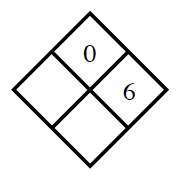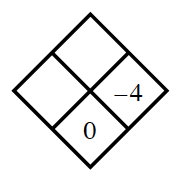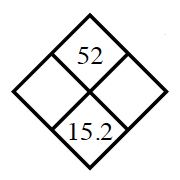### Home > CC2MN > Chapter 5 > Lesson 5.2.5 > Problem5-75

5-75.

Copy and complete each of the Diamond Problems below. The pattern used in the Diamond Problems is shown at right.1.What number multiplied by $6$ yields $0$?

Left: $0$
Bottom: $6$
Fill in the diamond.

1.Multiply the two given numbers for the top number. What do you do to the two given numbers to get the bottom number?

Top: $-16$
Bottom: $6$
Fill in the diamond.

1.The sum of what number and $-4$ is $0$?

What do you do to the left and right numbers to get the top number?

1.What numbers multiplied together give $52$?